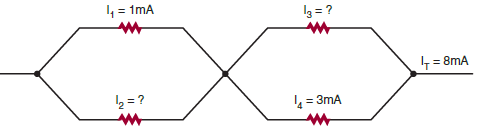# SSC JE Electrical Important MCQ Part 20

SSC JE Electrical Important MCQ PDF Part 20

1.What are the values of I₂ and I₃A.I₂=8 mA and I₃=0 mA

B.I₂=7 mA and I₃=5 mA

C.I₂=0 mA and I₃=8 mA

D.I₂=5 mA and I₃=7 mA

Answer  : B (Total Current It=8 mA,So I₂=It-I₁=8-1=7 mA, & I₃=It-I₄=8-3=5 mA)

2.Which symbol is not correctly matchedA.A
B.B
C.C
D.D

Answer  : D (D is the symbol of OHMMETER)

3.Which of following device use not correctly matched

A.DIODE-Rectification

B.ZENER DIODE-Voltage Regulation

C.DIAC-Choppers

D.SCR-Power Switching

Answer  : C (DIAC use for Trigger the circuit)

4.A power transformer has 1000 primary turns and 100 secondary turns. The cross-sectional area of the core is 6 sq. cm and the maximum flux density while in operation is 10 000 Gauss.What will be the turns per volt for the primary windings

A.133.2 V
B.13.32 V
C.7.5 V
D.10 V

(Given, N₁ = 1000; N₂ = 100; Ai = 6 cm² = 6 × 10⁻⁴ m² ,Bm = 10000 gauss = 1 tesla
So E₁ = 4.44 N₁f Bm Ai = 4.44 × 1000 × 50 × 1 × 6 × 10⁻⁴ = 133.2 V)

5.Stator core of a single-phase, split winding induction motor is made of

A.laminated cast iron

B.mild steel

C.silicon steel stampings

D.soft wood

6.In a single phase induction motor at start, the two revolving fields produce

A.unequal torques in the rotor conductors

B.no torque in the rotor conductors

C.equal and opposite torque in the rotor conductors

D.equal torques in same direction in the rotor conductors

7.During running condition if the starting winding of a split phase induction motor is disconnected

A.the motor will stop

B.the motor winding will burn

C.the main winding will be damaged

D.the motor will continue to rotate

8.The function of the centrifugal switch is to disconnect the

A.main winding during starting

B.auxiliary winding during starting

C.main winding during run.

C.auxciliary winding during running

9.Which motor is best suited for domestic refrigerator

A.A3-phase induction motor

B.Universal motor

C.Capacitor start motor

10.The material used for the construction of rotor of a hysteresis motor is

A.cast iron

B.copper

C.hardened high-retentivity steel

D.low-retentivity steel

11.The motors which are used in the control system are called

A.quick motors

B.servomotors

C.toy motors

D.all of these

Answer  : B (A servomotor is a rotary actuator or linear actuator that allows for precise control of angular or linear position, velocity and acceleration)

12.A 3-phase induction motor is called a 3-phase induction generator

A.when its speed is equal to synchronous speed

B.when it is driven at a speed higher than synchronous speed

C.when its slip is negative

D.both B and C

13.Usually, the normal value of the armature resistance of a DC motor is

A.100 Ω

B. 0.5 Ω

C.10 Ω

D.0.005 Ω

14.The ratio Eb/V of a DC motor represents its

A.efficiency

B.speed regulation

C.starting torque

D.Running Torque

Answer  : A (Eb-back emf or induced emf,V-supply voltage So Eb/V=Output/Input=Efficiency)

15.The power developed in the armature of a DC motor is equal to

A.armature current multiplied by back emf

B.power input minus losses

C.power output plus iron losses

C.power output multiplied by efficiency

Answer  : A (Eb Ia = Electrical equivalent of mechanical power (Pm) developed in the armature)

17.If the voltage applied across the armature of a DC shunt motor is increased by 5% keeping its load current and field constant, what will be the effect on its speed

A.decrease by about 5 per cent

B.remain unchanged

C.increase by about 5 per cent

D.increase by 10 per cent

(The speed of the DC motor can be given as N= k (Eb / ф )

Where, N = Speed of the motor

Eb= Back EMF of motor

ф = Field Flux

N ∝ Eb or V (Because others things are constant)

So if supply voltage increased by 5% then speed of the motor increased by 5%)

18.The torque available at the shaft of a DC motor is less than the torque developed in the armature because of………losses.

A.copper

B.mechanical

C.iron

D.rotational

19.The armature resistance of a 110 V DC motor is 0.5 ohm. What will be its armature current if the back emf is 100 V.

A.20 A

B.200 A

C.220 A

D.60 A

Answer  : A (Eb = V – IaRa, Ia=(V-Eb)/Ra=(110-100)/0.5=10/0.5=20 A)

20. With the increase in load, the speed of a DC shunt motor

A.reduces slightly

B.remains constant

C.increases slightly

D.increases proportionately